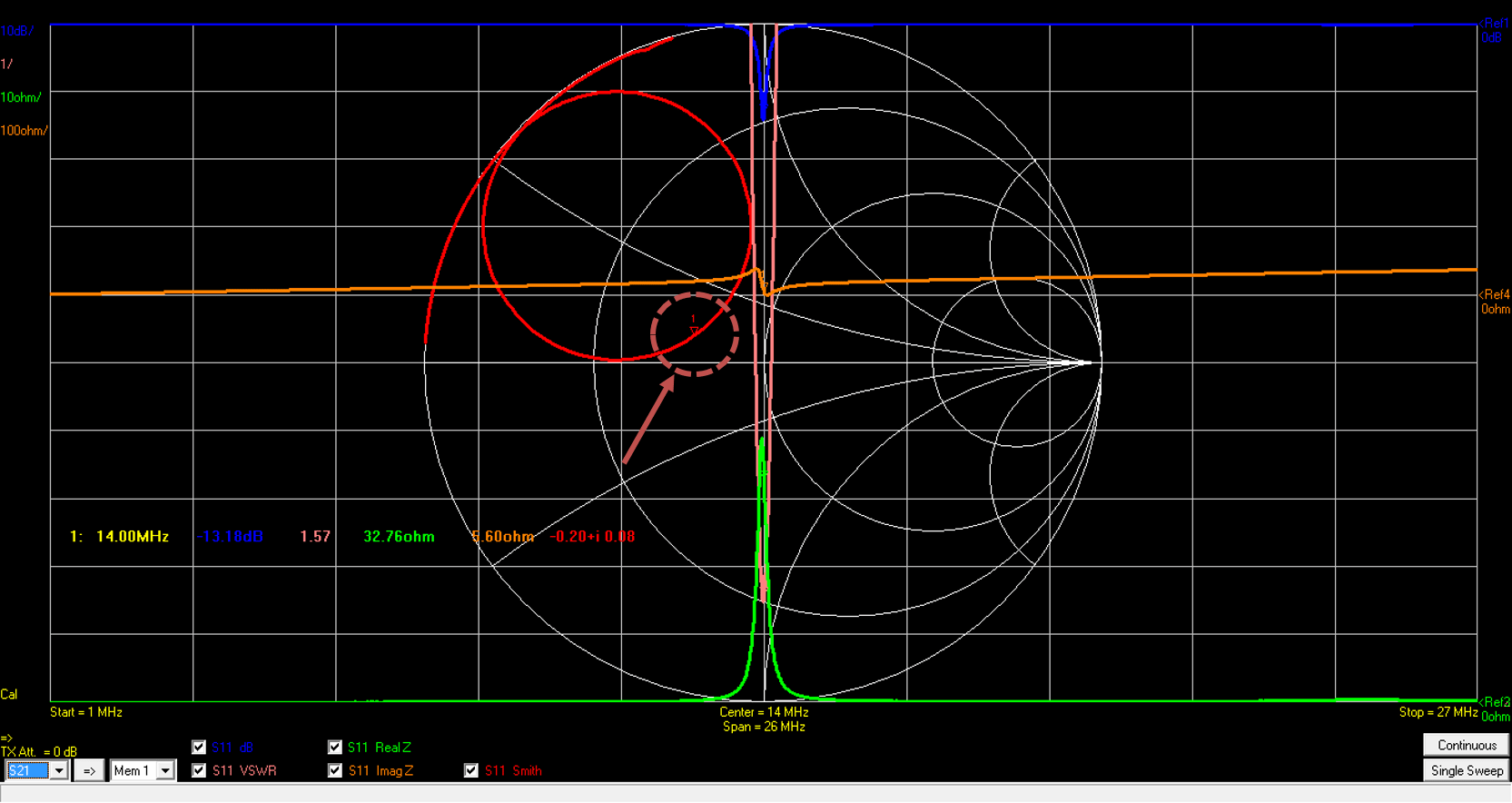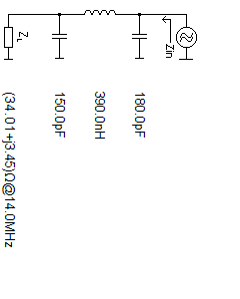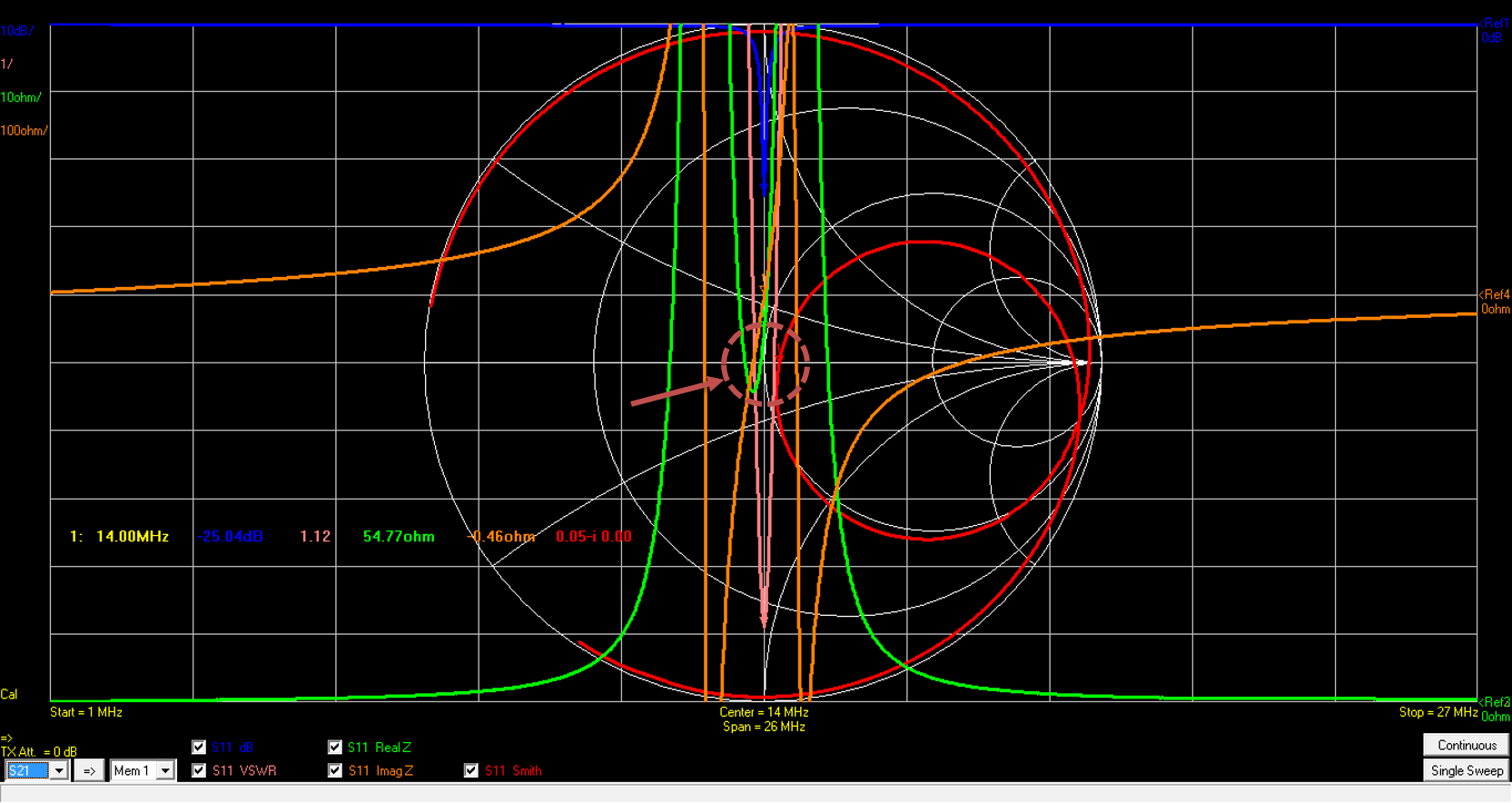Hello, I will explain how I matched input impedance of the WPT coil to 50 Ohm.

Please look at the following picture. You can see that SMA cable is directly connected with the feed coil.Let’s see the frequency characteristic properties of this WPT coil. Frequency range is between 1 MHz and 27 MHz. So the center frequency is 14 MHz (=0.5*(1+27) MHz) that is the resonant frequency of this WPT coil.

In the following picture, Blue line shows S11 [dB], Pink line shows VSWR, Green line shows Re(Zin), Yellow line shows Im(Zin) and Red line shows the Smith chart of S11.

We can easily find that the impedance is not matched at resonant frequency when we look at the cursor No.1 of the smith chart.

Its current S11 value at resonant frequency is -0.20 + j 0.08. We need to set this value close to 0.00 + j 0.00.

(Its current Zin value at resonant frequency is 32.76 + j 5.60 Ohm. We need to set this value close to 50 + j 0.0 Ohm.)Parameter Value Unit Frequency 14 MHz S11 -13.18 dB VSWR 1.57 Real Z 32.76 Ohm Imag Z 5.60 Ohm S11 -0.20 + j 0.08

Actually, I made 5 WTP coils and measured all S11 parameters and calculate the each average value.

When we look at the Zin value. the average value of Zin is 34.01 + j 3.45 OhmIn this experiments, We decide the PI network for matching impedance. With simple calculation, we extracted the required LC parameters like the following figure.

In this figure, ZL means the input impedance of coil.You can see how the impedance changes at PI network.Then, I built simple PCB board for PI network and put in the required circuit LC elements onto it and connected it between SMA cable and WPT coil like the following figure.OK, Let’see how the frequency response changes with the help of PI network. Wow. They look very different before impedance matching.

We can easily find that the cursor 1 get closer to 50 Ohm point of the smith chart. Very impressive.We can see how the S11 values are changed before and after impedance matching. It looks so nice and amazing. VSWR 1.12 looks really fantastic.

 Parameter Before Impedance matching After Impedance matching Unit Frequency 14 14 MHz S11 -13.18 25.04 dB VSWR 1.57 1.12 Real Z 32.76 54.77 Ohm Imag Z 5.60 -0.46 Ohm S11 -0.20 + j 0.08 -0.05 + j 0.00

## 1件のコメント

1. […]  http://wireless-square.com/2016/11/18/impedance-matching-of-the-wpt-coil/ […]

このサイトはスパムを低減するために Akismet を使っています。コメントデータの処理方法の詳細はこちらをご覧ください